Internal Cube FormulaOur first example involves calculating figures for Margin % in the Sales cube from Margin and Revenue values, also in the Sales cube. Proceed as follows:

To create a formula for Margin %:

1.   In the PowerOLAP ribbon, select Model tab and Cubes command.
The Cubes dialog box is opened.

2.   Select the Sales cube, then click the Formulas… button.
(Alternately, double-click once on the Sales cube.)
Under the last formula written in the Formula text box, press the Enter key to put a space between the last and the new formula to be written.TipYou may write a comment explaining what the formula is calculating. The comment can begin with two forward slashes (//), which will cause the formula editor to ignore everything that follows on that line. Alternatively, you can use a single forward slash followed by an asterisk (/*)—everything that follows (on consecutive lines) will be ignored up to the point where an asterisk, then forward slash (*/) conclude the comment.

The next step is to build the LHS of the formula, which will demonstrate use of the Build Range Reference dialog box.

3.   With the cursor on a new line in the Formula text box, click the Build Range Reference button  to define the LHS range reference. The Build Range Reference dialog box appears: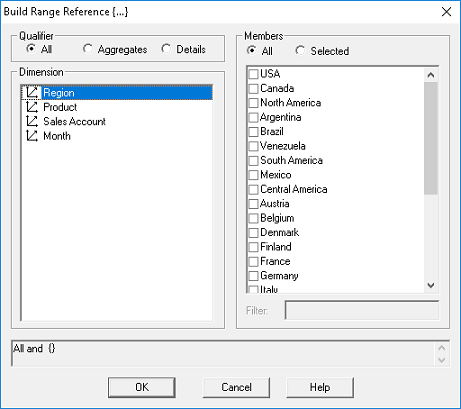4.   Select a Qualifier (in this case just select All).

Notice at the top left hand portion of the dialog the three radio buttons: All, Aggregates and Details. The qualifiers define in what intersections should the formula apply, whether it should apply to all, or just the aggregate points or detail points. Below is a description of what each option does.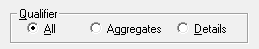Button Operator ALL Specifies that the LHS range includes all, i.e., both Aggregate and detail level, cells within the specified Member set(s). AGGREGATES Specifies that the LHS range includes only aggregate level cells within the specified Member set(s). DETAILS Specifies that LHS range includes only detail level cells within the specified Member set(s).

5.   Select a <Dimension> (in this case, Sales Account)
from the Dimension list box on the left.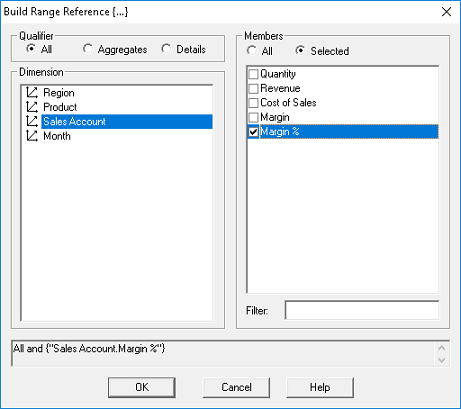On the right-hand side of the dialog box is a list of Member names whose values will be determined by the formula. Above the list, you can select the All radio button, in which case all Members will be affected; or, use the Selected button to enable the Filter text box below the list: you can enter a letter or a word to narrow the list of Members shown (helpful if it is a long list).

Proceed by selecting the member/s.

6.   Select Margin % from the Members list box on the right.

Notice at the bottom part of the dialog that it displays the formula expression that has been defined for the LHS.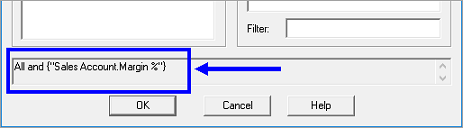7.   Click OK.

You are returned to the Formulas dialog box, which now displays the LHS of the formulas you just built, as in the example figure below (circled):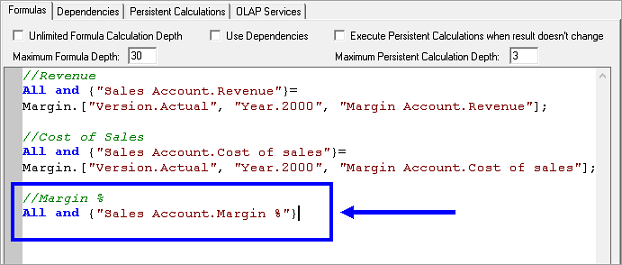[At this point, if you were simply making the LHS equal to a fixed number, you would type the “equals” sign and then the number.]

8.   Type = , or press thebutton, after the LHS of the formula.

Next step is to build the RHS (right-hand side) of the formula, using the Build Cube Reference dialog box.

9.   Select the Build Cube Reference button.
The Build Cube Reference dialog box appears.10. Select the <Cube> from the top drop-down menu, whose data you want to use (in this case, it is the same Cube, Sales—so Sales appears in the grayed-out Formula text area, at bottom).
[Note, if you selected another Cube, you would be building a cross-cube formula.]

11. Select a <Dimension> (in this case, again, it is Sales Account)
from the Dimension text box.

12. Select a <Member> from the Members text box for the first part of the equation
(in this case, Margin).

13. Click OK.

You are returned to the Formula text box, where you need to add the “divide by” sign (/) after the Margin reference: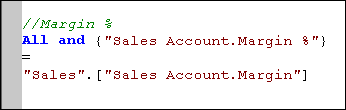TipYou can add a line break (Enter) anywhere in the formula text (as above, after the equals sign), to make the formula more legible—this has no bearing on the formula result.

14. Now you must finish the equation by building the last part of the statement so that Margin % = Margin/Revenue.

15. Again, click on the Build Cube Reference button and choose the Sales cube and Sales Account dimension.

16. Select the Member Revenue and click OK.

17. The RHS of the formula now must be completed by adding the semi-colon.

The completed formula appears as in the following figure: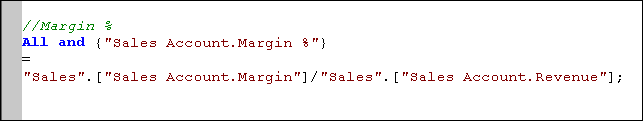18. Now click on the Check Syntax button in the Formulas dialog box to check the syntax of the formula for errors.
The following message box appears (against the background of the Formulas dialog box, as in the following figure):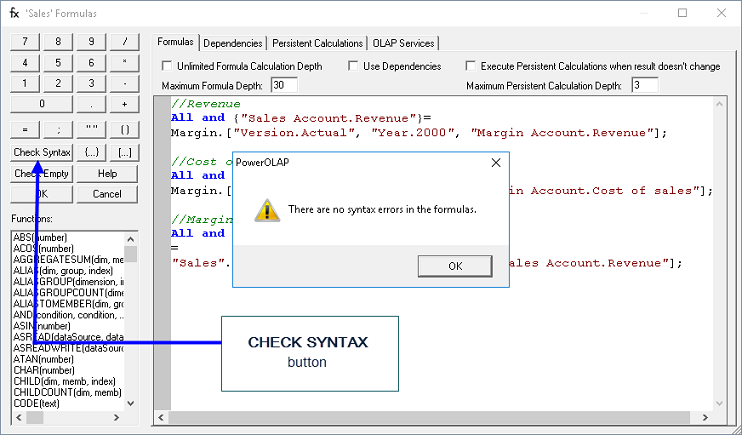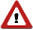WarningIf there is an error in the formula syntax, PowerOLAP® will return a message stating this, and a possible resolution (see example message box below).19. Click OK.

20. You are returned to the Cubes dialog box.
Click OK to exit the dialog box.

If at this point you create a slice out of the Sales cube and refresh to update values, you will see that some cells have correctly calculated Margin % value, while others return an error—#DIV/0 (a detail of which is shown boxed in the following figure) —signifying that those values could not compute because there are zero values in the divisor of the formula.IF Statement

In this next topic, we will discuss how to create an IF statement in PowerOLAP. In the previous topic, we have noted that the slice returned some error values (i.e., #DIV/0 errors). These errors resulted from trying to divide a certain number or value by zero, which then will yield an undefined result.

To resolve those errors, as in the example above, you can define an IF statement to set a condition that if the divisor is empty, then force the result to become a certain number, text or value. In the demonstration below, an IF statement will be created to set the result to zero if the divisor is zero.

1.   To create the IF Statement, go to the Cubes dialog and return to the Formula Editor dialog box for Sales cube.

2.   Go to the formula for calculating the Margin % and place your cursor just after the “equals” sign, i.e., in front of the RHS of the equation:3.   We can use the IF statement pre-formatted for us in the Functions list box of the Formula Editor on the left by double clicking on the function, which brings it into our Formula text, or you may simply type in directly the IF expression: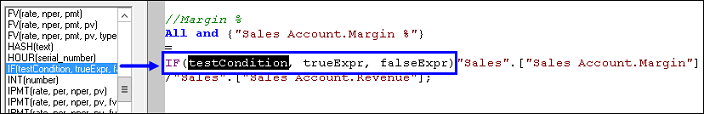In fact, part of our statement is already written for us in the formula, as you will see. The important thing to note is that the IF function guides us in the construction of our statement, which, in plain English would be: “If Revenue equals zero, then return the value zero; otherwise perform the calculation Margin/Revenue.”

Proceed to writing the IF Statement.

4.   First define the test Condition by highlighting the word testCondition and replace it with the appropriate conditional statement —i.e., Revenue = 0.

5.   To create the first part of our statement
(the “testCondition”, meaning “if Revenue equals zero”), first build the reference to Revenue by clicking on the Build Cube Reference button.
From the Sales cube, choose the member Revenue from the Sales Account dimension. Click OK.
Then add =0, (don’t forget the comma after the zero).

The “testCondition” part of the equation now appears like this (circled in the following figure):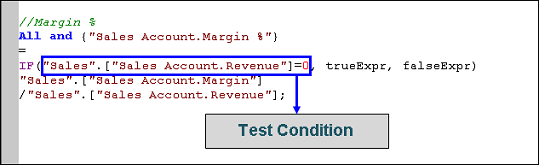6.   Next, create the “trueExpr” (“then return the value zero”),
highlight  the word and simply type 0, (don’t forget the comma).

7.   Next, create the “falseExpr” (“otherwise return the calculation Margin/Revenue“)—Since this already exists as the formula entered earlier in the exercise, simply higlight the formula, then paste it over the parameter “falseExpr”.

8.   Next, make sure that your formula is ended with a semi-colon ( ; ).

In the example, we just left the semi-colon outside the IF expression. The formula should look as follows:9.   Click the Check Syntax button and click OK.

Now when you recalculate the Sales slice, the formula (which includes the IF statement) works correctly, and zeros appear in the cells where Revenue equals zero.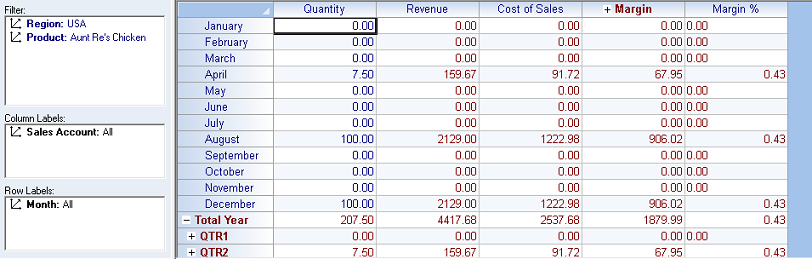NotesAt this point, you can delete the formula, copy it to a new location (e.g., a text file), or surround it with forward-slash, asterisk (concluding with asterisk, forward-slash) in order to proceed with the following example, also a calculation of Margin %, but this time the result of a cross-cube formula.